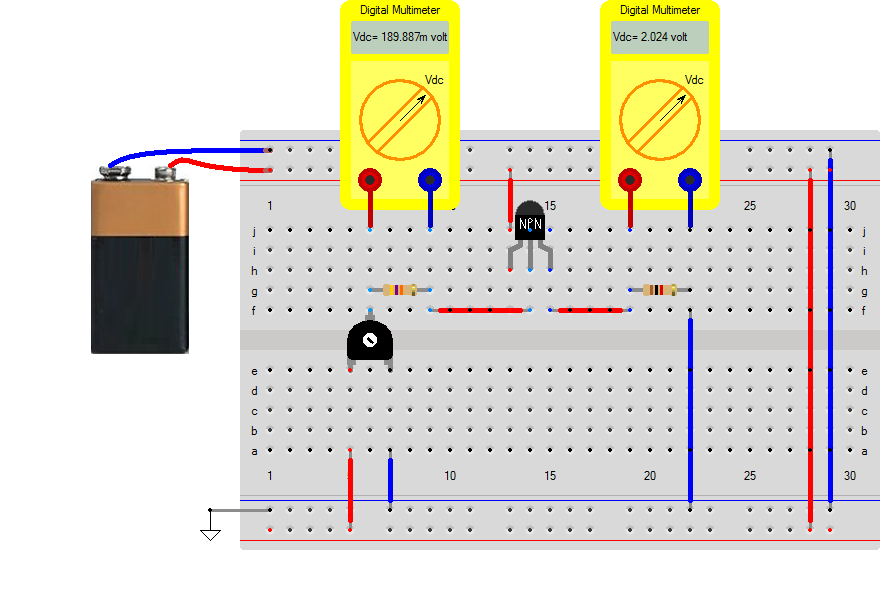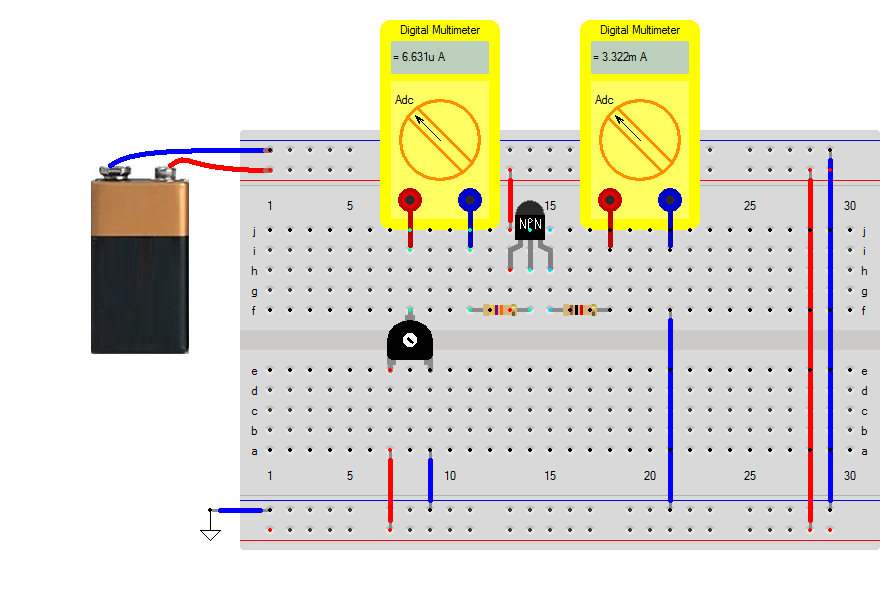• Introduction
• Power Electronics
• SMPS
• DC Drives
• AC Drives
• Drives
• Green Energy

## Bipolar Transistors

The bipolar transistor comes in two variant for the breadboard, as npn and pnp. Both have a gain of $$H_{fe} = 500$$ and the base-emittor voltage drop is around $$0.7 volt$$. The voltage over the collector-emittor $$V_{ce}$$ depends on how the transistor is driven and on how deep the transistor is saturated.

### Emittor Follower

The common collector circuit is often referred to as an emitter follower circuit. Why this is, you learn when you look at the voltages in a common collector circuit.

The emittor follower has a resistor of $$R=1k\Omega$$ between emittor and battery-minus and the collector is directly connected to the battery-plus. A variable resistor of $$R=100k\Omega$$ in series with a resistor of $$R=47k\Omega$$ is connected between the battery-[plus and the base of the transistor. Since the potentiometer is connected between the plus and minus terminal of the battery, we can create a voltage between 0 and 9 volt.

Using the variable resistance the current to the base can be varied, by changing the parameter position between [0 .. 1]. This parameter can be changed when you click with the right-mouse button on the variable resistor.

Base current and emittor current are measured as voltage of the resistors. Note that the emittor current $$I_e$$ is a summation of collector current $$I_c = H_{fe} \cdot I_b$$ and base current $$I_b$$ $I_e = I_b + H_{fe} \cdot I_b$From the simulation we read a current of 2mA through the resistor of $$R=1k\Omega$$. This means that the base current will be $I_b=\frac{2mA}{500} = 4 \mu A$

The voltage on the base of the transistor will be the voltage over the resistor (measured with the DMM), plus the voltage drop $$V_{be}$$ and will equal to $V_b = 2 + 0.7 = 2.7 volts$

The voltage over the base resistor (measured with the DMM), is equal to $V_{47k\Omega} = 47k \cdot 4\mu A = 188mV$ Adding up, this will give us around 3volt at the variable terminal of the potentiometer

The potentiometer is is fixed to the position of 0.66. This means that the voltage in the ideal case is 3 volt. Putting all in an equation gives $I_b= \frac{V_{potentiometer} - V_{be} - V_{[R=1k]}\cdot I_c}{47k}$ with $I_c = H_{fe} \cdot I_b$ Entering the values gives $I_b= \frac{3 - 0.7 - 1k\cdot H_{fe} \cdot I_b}{47k}$ rearranging $47k \cdot I_b= 2.3 - 1k\cdot 500 \cdot I_b$ gives$I_b= \frac{2.3}{47k+500k} = 4.2 \mu A$

### Emittor Follower

To see why the circuit is called an emittor follower, change the value of the potentiometer to 0.5. This means that the voltage at the potentiometer will be approximately 4.5 volt. Lets again calculate base and emittor currents using $I_b= \frac{V_{potentiometer} - V_{be} - V_{[R=1k]}\cdot I_c}{47k}$ with $I_c = H_{fe} \cdot I_b$ Entering the values gives $I_b= \frac{4.5 - 0.7 - 1k\cdot H_{fe} \cdot I_b}{47k}$ rearranging $47k \cdot I_b= 3.8 - 1k\cdot 500 \cdot I_b$ gives$I_b= \frac{3.8}{47k+500k} = 6.9 \mu A$ and the collector current is $I_c = H_{fe} I_b = 500 \cdot 6.9 \mu A = 3.45mA$### Common Collector

Instead of using the DMM-Vdc we can also use the DMM-Adc to directly measure the currents. By measuring the currents, we can see that the above equations are not exact. Why is this? Notice that the voltage for driving the base is build using the potentiometer which has an internal resistance of 100kΩ. This means that a current of 6μA to 7μA causes an extra voltage drop that will eventually lower everything. Measure the voltage at the potentiometer and redo the math so see if this is true.Move the mouse pointer over the nodes in the breadboard and you will measure around 4.33 volt at the potentiometer. $I_b= \frac{V_{potentiometer} - V_{be} - V_{[R=1k]}\cdot I_c}{47k}$ with $I_c = H_{fe} \cdot I_b$ Entering the values gives $I_b= \frac{4.33 - 0.7 - 1k\cdot H_{fe} \cdot I_b}{47k}$ rearranging $47k \cdot I_b= 3.63 - 1k\cdot 500 \cdot I_b$ gives$I_b= \frac{3.63}{47k+500k} = 6.63 \mu A$ and the collector current is $I_c = H_{fe} I_b = 500 \cdot 6.6 \mu A = 3.32mA$